Question

# A juggler tosses and catches balls at waist level. The balls are tossed at launch angles...

A juggler tosses and catches balls at waist level. The balls are tossed at launch angles of 66°. If a ball attains a height 64 cm above waist level, how long is a ball in the air? In Seconds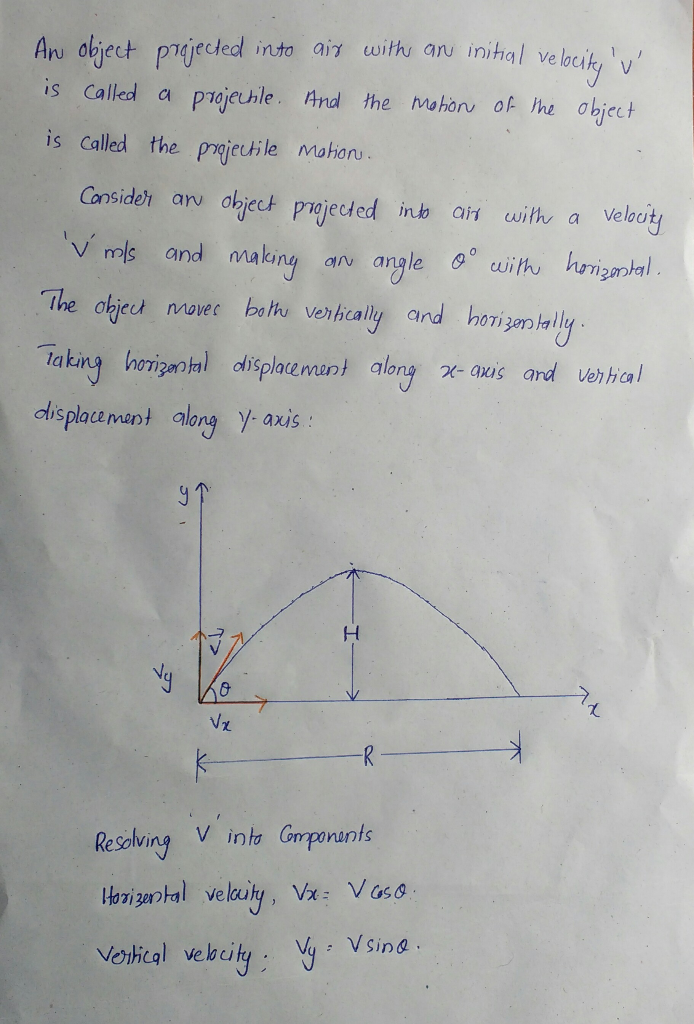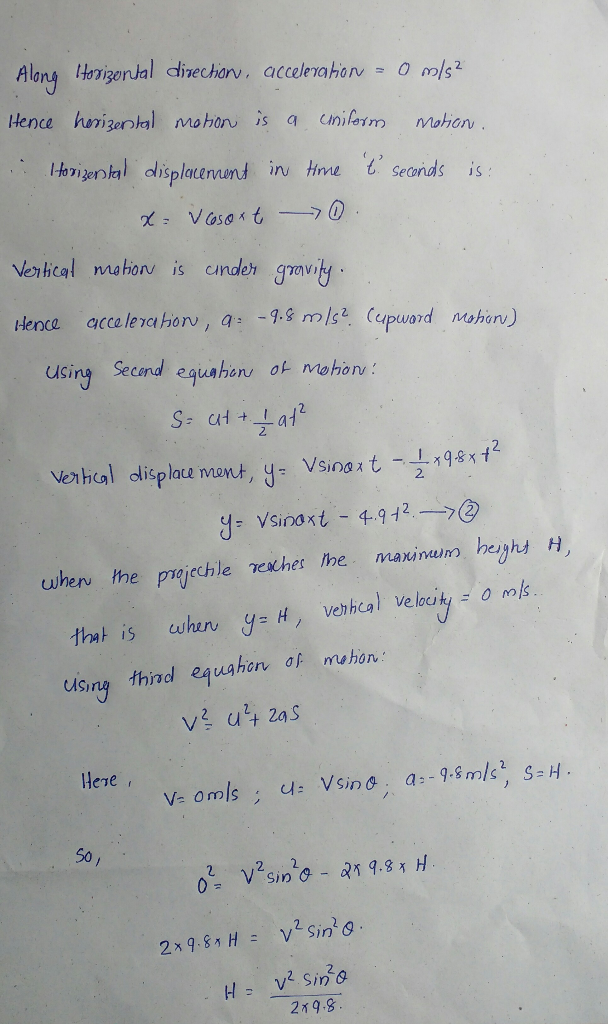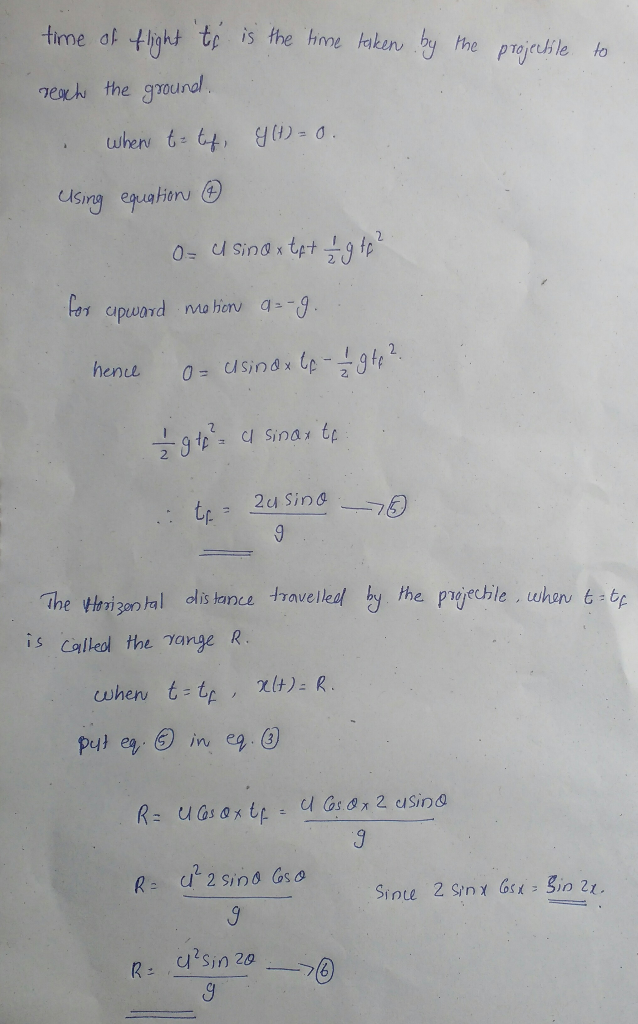in projectile motion the equation for maximum height is given by the equation

H = u2 Sin2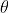/ 2g

given H = 64 cm = 0.64 m

g = 9.8 m/s2

u2 Sin2= 2gH

u Sin= (2gh)1/2

u Sin= (2 x 9.8 x 0.64)1/2 = 3.54 m/s

equation for time of flight

T = 2 u Sin/ g = 2 x 3.54 /9.8 = 0.722 s

#### Earn Coins

Coins can be redeemed for fabulous gifts.

Similar Homework Help Questions
• ### A second baseman tosses the ball to the first baseman, who catches it at the same...

A second baseman tosses the ball to the first baseman, who catches it at the same level from which it was thrown. The throw is made with an initial speed of 19.0 m/s at an angle of 35.0 degrees above the horizontal. How long, in seconds, is the ball in the air? give me in detail

• ### 2. An aspiring juggler realizes that the acceleration due to gravity can be measured simply by...

2. An aspiring juggler realizes that the acceleration due to gravity can be measured simply by comparing the time interval an object spends above a certain height when tossed vertically upwards to the total time it is in the air. Suppose she tosses a small ball vertically upwards and catches it a moment later (after a time ∆T elapses) at the same height it was released. In flight, the ball spends a time interval ∆t above a certain height H...

• ### A second baseman tosses the ball to the first baseman, who catches it at the same...

A second baseman tosses the ball to the first baseman, who catches it at the same level from which it was thrown. The throw is made with an initial speed of 19.0 m/s at an angle of 33.0 ∘above the horizontal. Part a) What is the horizontal component of the ball's velocity just before it is caught? Part b) How long is the ball in the air?

• ### A juggler performs in a room whose ceiling is a height 2.5 m above the level...

A juggler performs in a room whose ceiling is a height 2.5 m above the level of his hands. He throws a ball upward so that it just reaches the ceiling. a)What is the initial velocity of the ball? b)What is the time required for the ball to reach the ceiling? c)At the instant when the first ball is at the ceiling, he throws a second ball upward with two-thirds the initial velocity of the first. How long after the...

• ### A ball is launched directly upward from ground level with an initial speed of 22 m/s....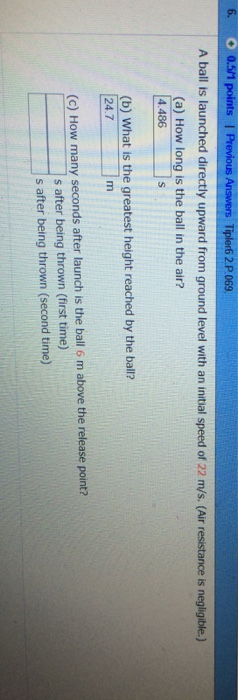A ball is launched directly upward from ground level with an initial speed of 22 m/s. (Air resistance is negligible) How long is the bail in the air? 4.486s What ls the greatest height reached by the ball? 24.7m How many seconds after launch is the ball 6 m above the release point? s after being thrown (first time) s after being thrown (second time)

• ### A ball is launched directly upward from ground level with an initial speed of 22 m/s....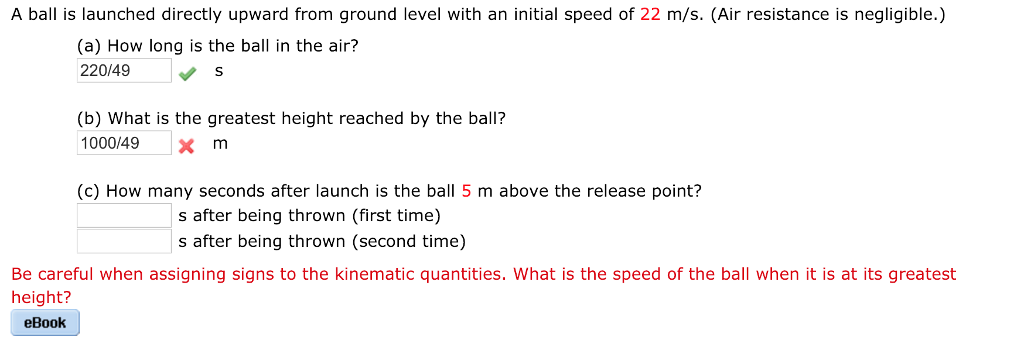A ball is launched directly upward from ground level with an initial speed of 22 m/s. (Air resistance is negligible.) (a) How long is the ball in the air? 220/49 S (b) What is the greatest height reached by the ball? 1000149xm (c) How many seconds after launch is the ball 5 m above the release point? s after being thrown (first time) s after being thrown (second time) Be careful when assigning signs to the kinematic quantities. What is...

• ### A ball is launched directly upward from ground level with an initial speed of 18 m/s....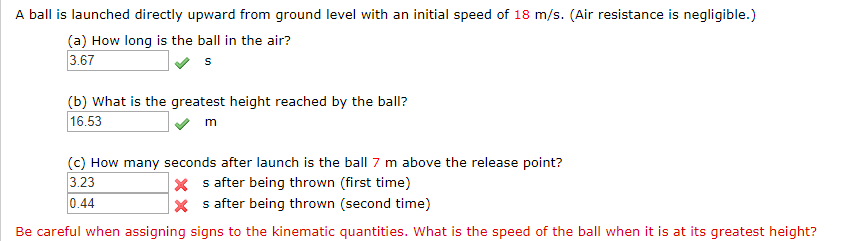A ball is launched directly upward from ground level with an initial speed of 18 m/s. (Air resistance is negligible.) (a) How long is the ball in the air? 3.67 (b) What is the greatest height reached by the balil? 16.53 (c) How many seconds after launch is the ball 7 m above the release point? 3.23 x s after being thrown (first time) x s after being thrown (second time) 0.44 Be careful when assigning signs to the kinematic...

• ### Two Thrown Balls 1 2 3 45678 A blue ball is thrown upward with an initial...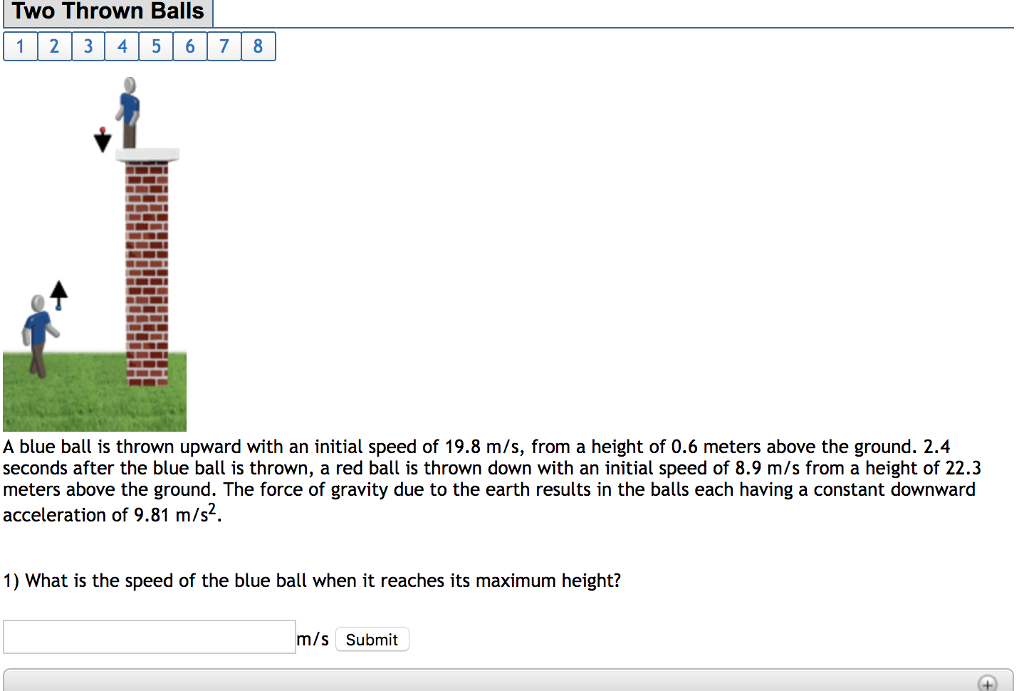Two Thrown Balls 1 2 3 45678 A blue ball is thrown upward with an initial speed of 19.8 m/s, from a height of 0.6 meters above the ground. 2.4 seconds after the blue ball is thrown, a red ball is thrown down with an initial speed of 8.9 m/s from a height of 22.3 meters above the ground. The force of gravity due to the earth results in the bals each having a constant downward acceleration of 9.81 m/s2....

• ### A red ball is thrown down with an initial speed of 1.3 m/s from a height...

A red ball is thrown down with an initial speed of 1.3 m/s from a height of 26 meters above the ground. Then, 0.4 seconds after the red ball is thrown, a blue ball is thrown upward with an initial speed of 23.9 m/s, from a height of 1 meters above the ground. The force of gravity due to the earth results in the balls each having a constant downward acceleration of 9.81 m/s2. What is the speed of the...

• ### A blue ball is thrown upward with an initial speed of 24.1 m/s, from a height...

A blue ball is thrown upward with an initial speed of 24.1 m/s, from a height of 0.8 meters above the ground. 2.9 seconds after the blue ball is thrown, a red ball is thrown down with an initial speed of 10.4 m/s from a height of 31.4 meters above the ground. The force of gravity due to the earth results in the balls each having a constant downward acceleration of 9.81 m/s2. What is the height of the red...